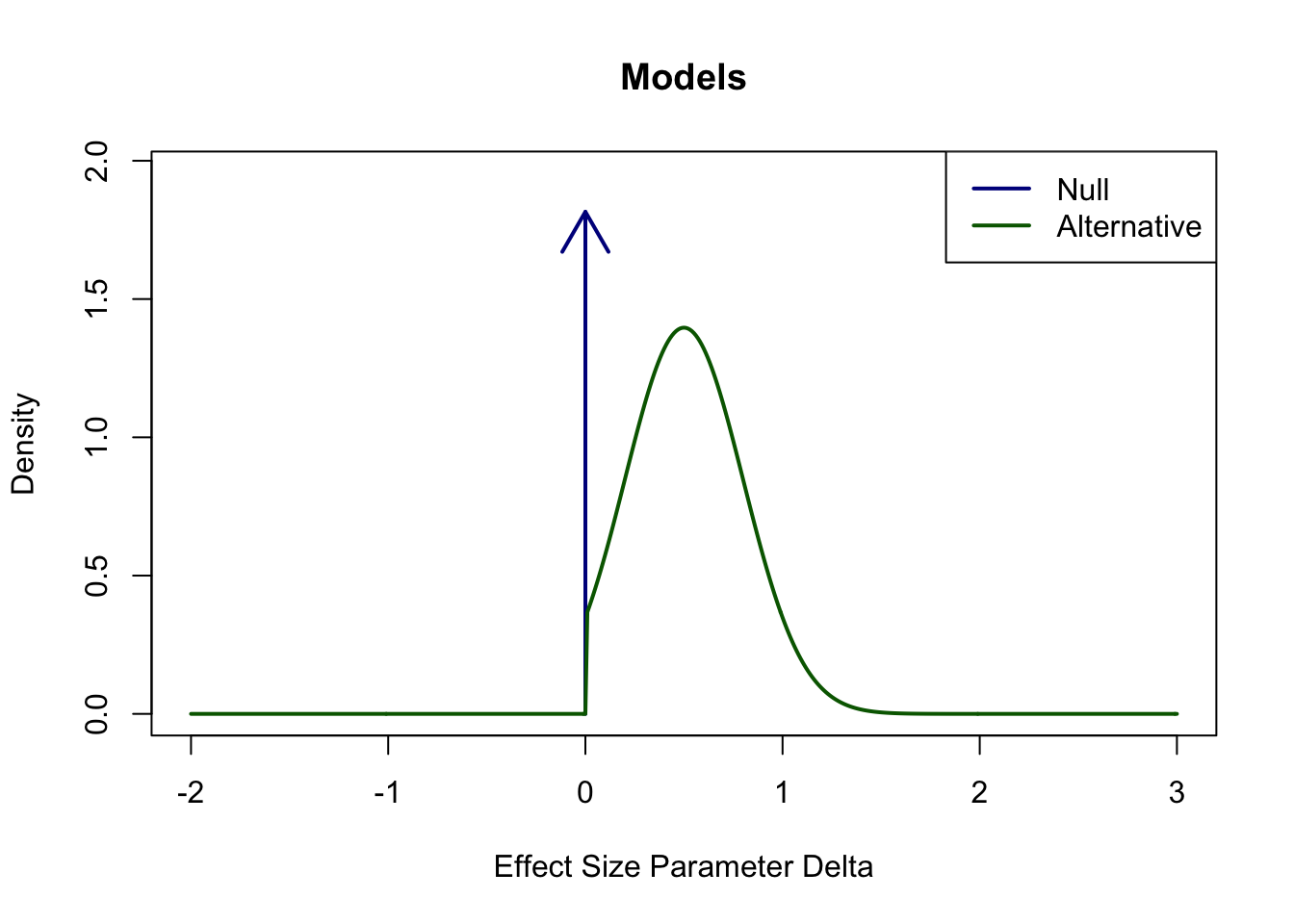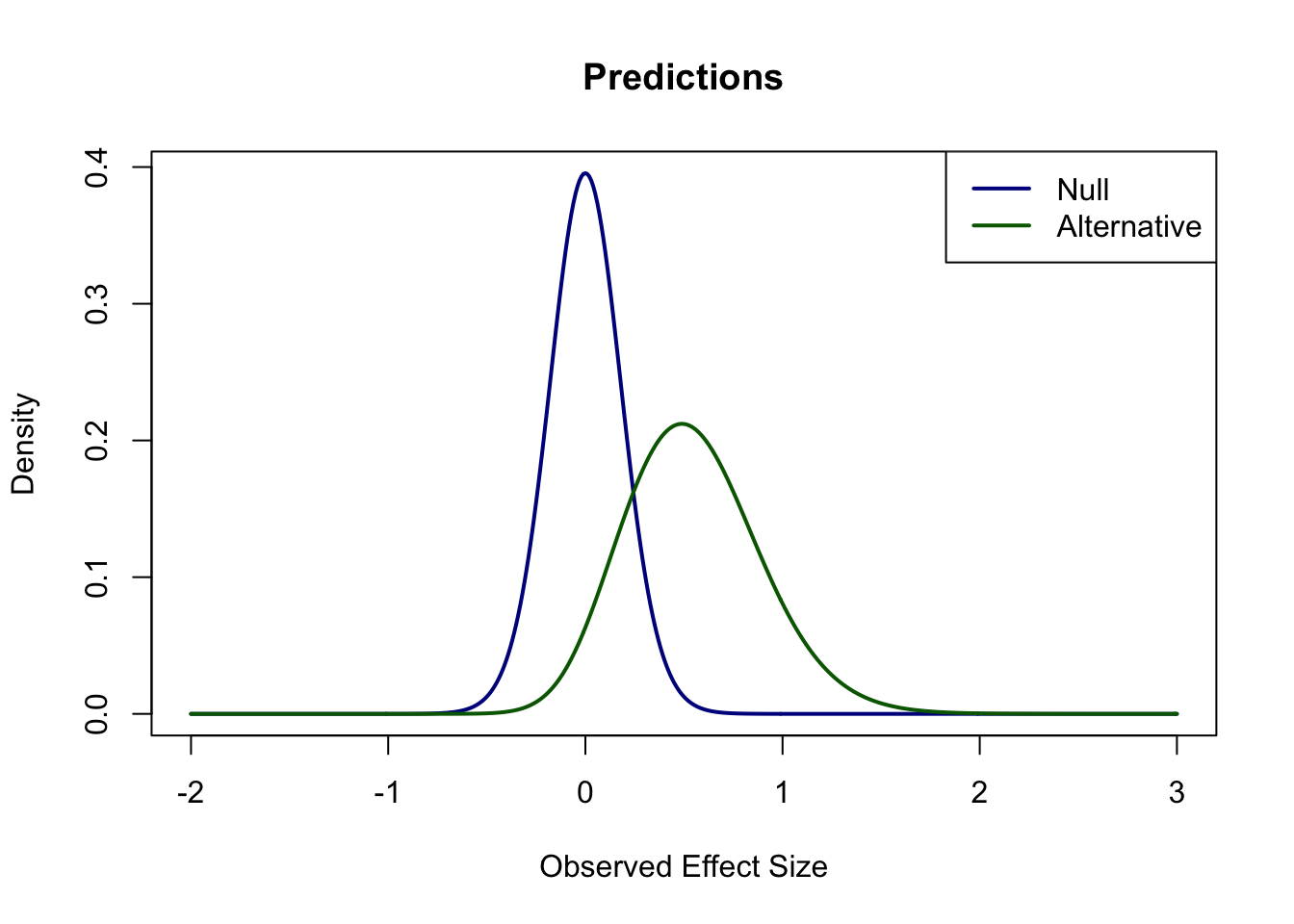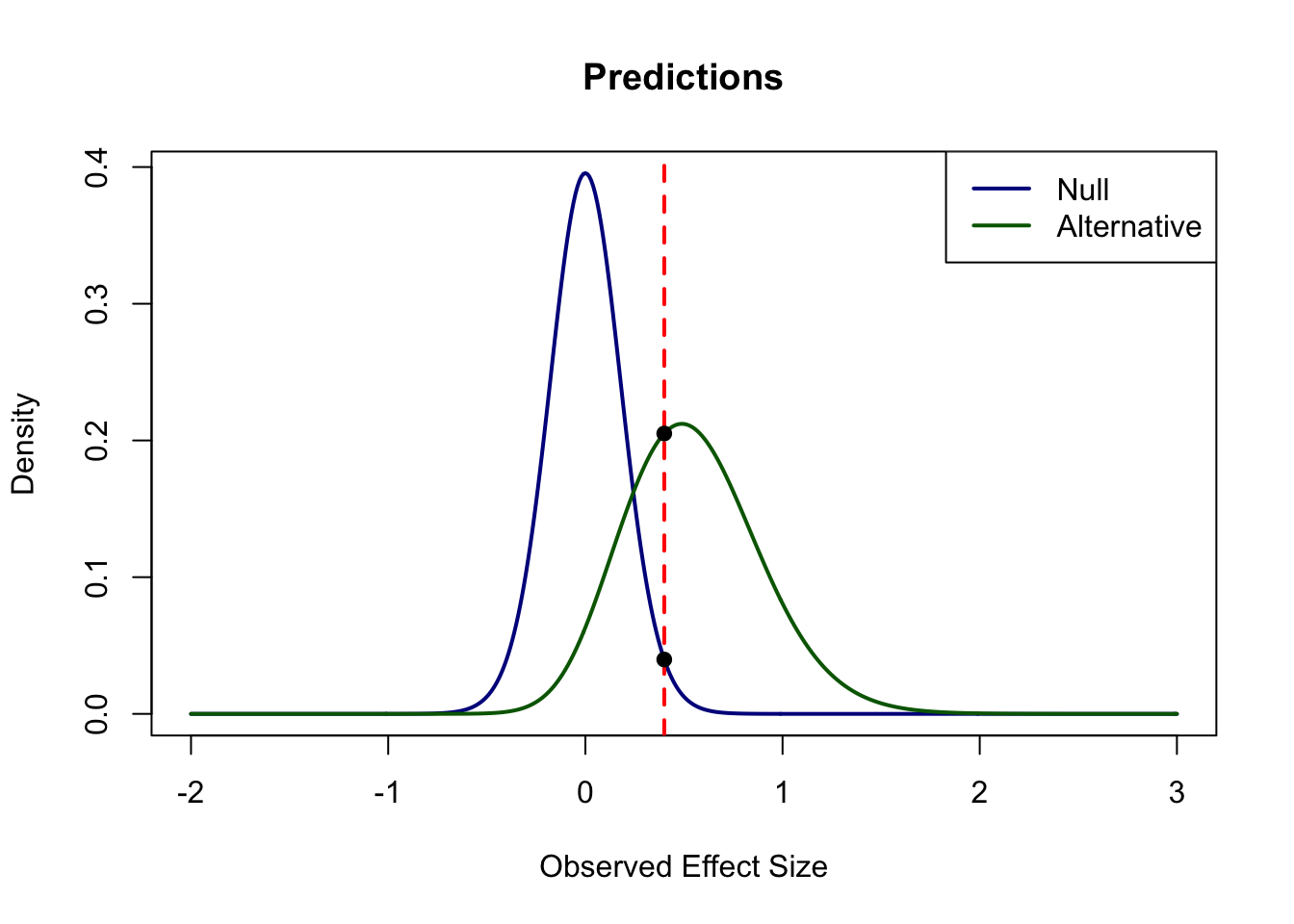## Sunday, January 24, 2016

### Roll Your Own: How to Compute Bayes Factors For Your Priors

Sometimes people ask what prior they should use in computing Bayes factor. It’s a great question, and trying to answer it leads to a deeper understanding of Bayesian methods.  Here I provide some R code so that you can visualize and compute Bayes factors for (almost) any prior you wish. At the end of this blog I provide all the R code in one block. You might even want to go down there and cut-and-paste it into your R editor now.

The Bayes factor compares two models. Let’s take one of them to be a point null. The other, the alternative, is up to you.

### Models

Let’s first start with a data model. Data are independent normal draws with mean μ and variance σ2. It is more convenient re-express the normal as a function of effect size, δ and σ2 where δ = μ/σ). Here is the formal specification:
$$Y_i \mid \delta,\sigma^2 \stackrel{iid}{\sim} \mbox{Normal}(\sigma\delta,\sigma^2).$$

A null model is implemented by setting δ = 0. The alternative model on effect size is up to you. Here is how you do it. Below is the specification for the R function altDens, the density of the alternative. I have chosen a normal with mean of .5 and a standard deviation of .3. I have also truncated this distribution at zero, and negative values are not allowed. You can see that in the specification of the function altDens. Set lo and hi, the support of the alternative. Here I set them to 0 and infinity, respectively.
#Specify Alternative (up to constant of propertionality)
lo=0 #lower bound of support
hi=Inf #upper bound of support
altDens=function(delta)
dnorm(delta,.5,.3)*as.integer(delta>lo)*as.integer(delta<hi)
You may notice that this alternative is not quite a proper density because it does not integrate to 1 That’s ok, the following function scales the alternative so the density does indeed integrate to 1.0.
#Normalize alternative density in case user does not,
K=1/integrate(altDens,lower=lo,upper=hi)$value f=function(delta) K*altDens(delta) ### Visualizing the Models Let’s now take a look at the competing models on δ, the effect size. Here is the code and the graphs: delta=seq(-2,3,.01) maxAlt=max(f(delta)) plot(delta,f(delta),typ='n',xlab="Effect Size Parameter Delta",ylab="Density",ylim=c(0,1.4*maxAlt),main="Models") arrows(0,0,0,1.3*maxAlt,col='darkblue',lwd=2) lines(delta,f(delta),col='darkgreen',lwd=2) legend("topright",legend=c("Null","Alternative"),col=c('darkblue','darkgreen'),lwd=2)### Bayes Factor Computation Bayes factor is based on prediction. It is the ratio of the predicted density of the data under one model relative to that under another. The predicted density of data for the null is easy to compute and is related to the central t distribution. Here is the code. nullPredF=function(obs,N) dt(sqrt(N)*obs,N-1) We can compute this predicted density for any observed effect size or for all of them. The following code does it for a reasonable range of effect sizes for a sample size of 30. You can change N, the sample size, as needed. obs=seq(-2,3,.01) N=30 nullPred=nullPredF(obs,N) Getting the predictive density for the alternative is a bit harder. For each nonzero effect size parameter, the distribution of the observed effect follows a noncentral t distribution. Hence, to obtain predictions across all nonzero effect size parameters, we need to integrate the alternative model against the noncentral t distribution. Here is the code with a simple loop: altPredIntegrand=function(delta,obs,N) dt(sqrt(N)*obs,N-1,sqrt(N)*delta)*f(delta) altPredF=function(obs,N) integrate(altPredIntegrand,lower=lo,upper=hi,obs=obs,N=N)$value

I=length(obs)
altPred=1:I
for (i in 1:I) altPred[i]=altPredF(obs[i],N)
Now we can plot the predictions for all observed effect sizes:
top=max(altPred,nullPred)
plot(type='l',obs,nullPred,ylim=c(0,top),xlab="Observed Effect Size",ylab="Density",main="Predictions",col='darkblue',lwd=2)
lines(obs,altPred,col='darkgreen',lwd=2)
legend("topright",legend=c("Null","Alternative"),col=c('darkblue','darkgreen'),lwd=2)### Let’s Run an Experiment

Suppose we just ran an experiment and observed a sample effect size of .4. Let’s look at the predictions for this value.
plot(type='l',obs,nullPred,ylim=c(0,top),xlab="Observed Effect Size",ylab="Density",main="Predictions",col='darkblue',lwd=2)
lines(obs,altPred,col='darkgreen',lwd=2)
legend("topright",legend=c("Null","Alternative"),col=c('darkblue','darkgreen'),lwd=2)

my.es=.4
abline(v=my.es,lty=2,lwd=2,col='red')
valNull=nullPredF(my.es,N)
valAlt=altPredF(my.es,N)
points(pch=19,c(my.es,my.es),c(valNull,valAlt))#cat("Predictive Density under the null is ",valNull)
#cat("Predictive Density under the specified alternative is ",valAlt)
#cat("Bayes factor (alt/null) is ",valAlt/valNull)
How well did the models do? Well, this value was clearly better predicted under the specified alternative than under the null. The density under the null is .04; the density under the alternative .205, and the ratio between them is 5.16-to-1. This ratio is the Bayes factor. In this case the Bayes factor indicate support of slightly more than 5-to-1 for the specified alternative.

### Other Alternatives

The alternative is specified with just a bit of R code that can be changed. Suppose, for example, one wished a flat or uniform alternative from -.2 to 1.2. Here is the bit of code
#Specify Alternative (up to constant of propertionality)
lo=-.2 #lower bound of support
hi=1.2 #upper bound of support
altDens=function(delta)
dunif(delta,lo,hi)
Run this first, and then run the rest to see what happens.
Here is another specification; this alternative is a gamma
#Specify Alternative (up to constant of propertionality)
lo=0 #lower bound of support
hi=Inf #upper bound of support
altDens=function(delta)
dgamma(delta,shape=2,scale=.5)

### The R Code in One Block

N=30
my.es=.4

############################
#Specify Alternative (up to constant of propertionality)
#Change this section to change alternative
lo=0 #lower bound of support
hi=Inf #upper bound of support
altDens=function(delta)
dnorm(delta,.5,.3)*as.integer(delta>lo)*as.integer(delta<hi)
###########################

#Normalize alternative density in case user does not,
K=1/integrate(altDens,lower=lo,upper=hi)$value f=function(delta) K*altDens(delta) delta=seq(-2,3,.01) #Plot Alternative as a density and Null as a point arrow maxAlt=max(f(delta)) plot(delta,f(delta),typ='n',xlab="Effect Size Parameter Delta",ylab="Density",ylim=c(0,1.4*maxAlt),main="Models") arrows(0,0,0,1.3*maxAlt,col='darkblue',lwd=2) lines(delta,f(delta),col='darkgreen',lwd=2) legend("topright",legend=c("Null","Alternative"),col=c('darkblue','darkgreen'),lwd=2) #Prediction Function Under Null nullPredF=function(obs,N) dt(sqrt(N)*obs,N-1) #Prediction Function Under the Alternative altPredIntegrand=function(delta,obs,N) dt(sqrt(N)*obs,N-1,sqrt(N)*delta)*f(delta) altPredF=function(obs,N) integrate(altPredIntegrand,lower=lo,upper=hi,obs=obs,N=N)$value

obs=delta
I=length(obs)
nullPred=nullPredF(obs,N)
altPred=1:I
for (i in 1:I) altPred[i]=altPredF(obs[i],N)

#Plot The Predictions
top=max(altPred,nullPred)
plot(type='l',obs,nullPred,ylim=c(0,top),xlab="Observed Effect Size",ylab="Density",main="Predictions",col='darkblue',lwd=2)
lines(obs,altPred,col='darkgreen',lwd=2)
legend("topright",legend=c("Null","Alternative"),col=c('darkblue','darkgreen'),lwd=2)

#Evaluate Predicted Density at Observed Value my.es
abline(v=my.es,lty=2,lwd=2,col='red')
valNull=nullPredF(my.es,N)
valAlt=altPredF(my.es,N)
points(pch=19,c(my.es,my.es),c(valNull,valAlt))
cat("Bayes factor (alt/null) is ",valAlt/valNull)

#### 1 comment:

Jessy Ryder said...

Most employers require a masters or a management degree for a top job placement, as a result the need for an MBA is essential in landing a lucrative job. science homework help online